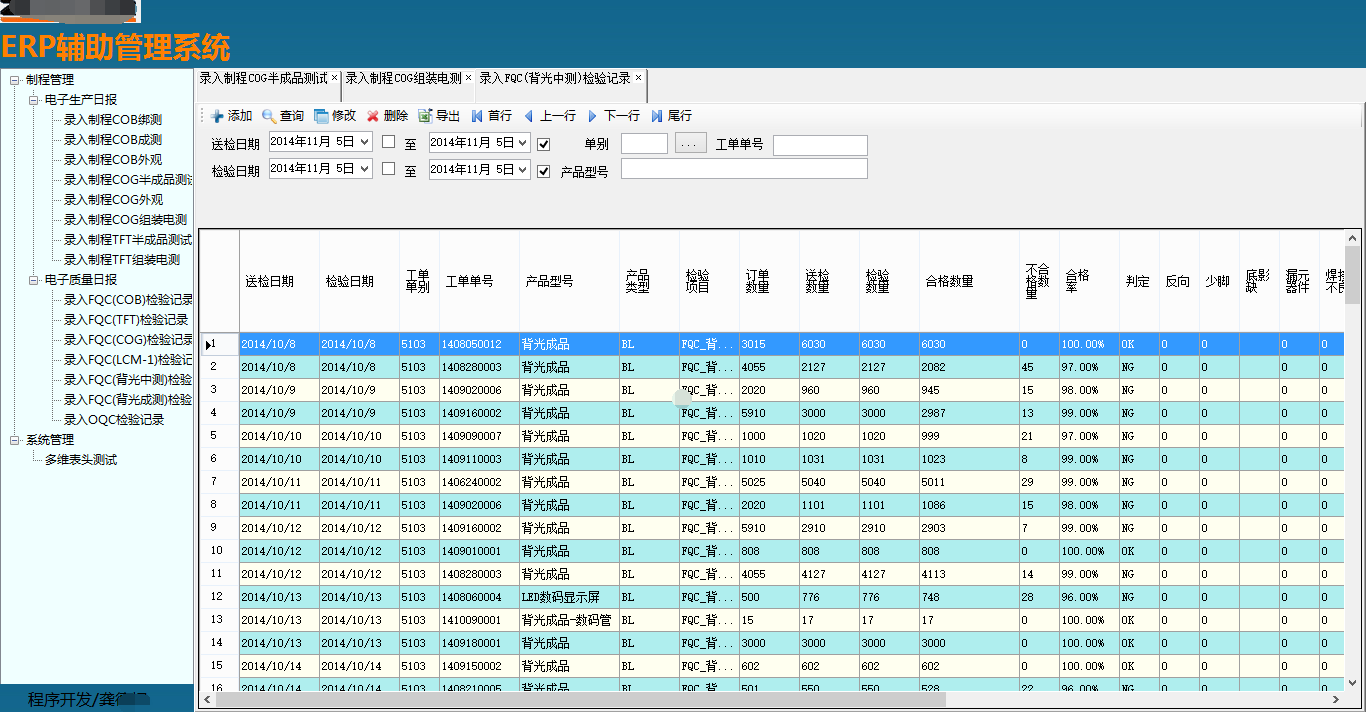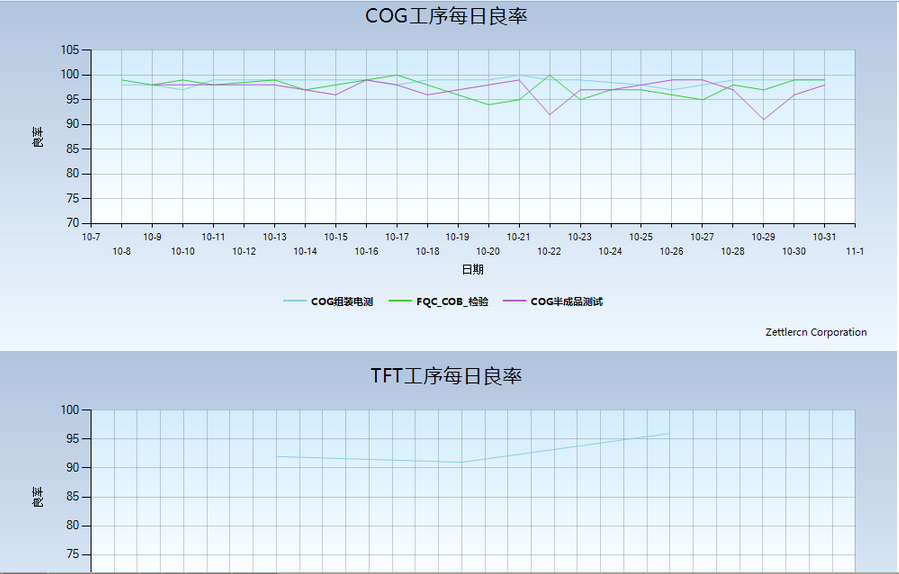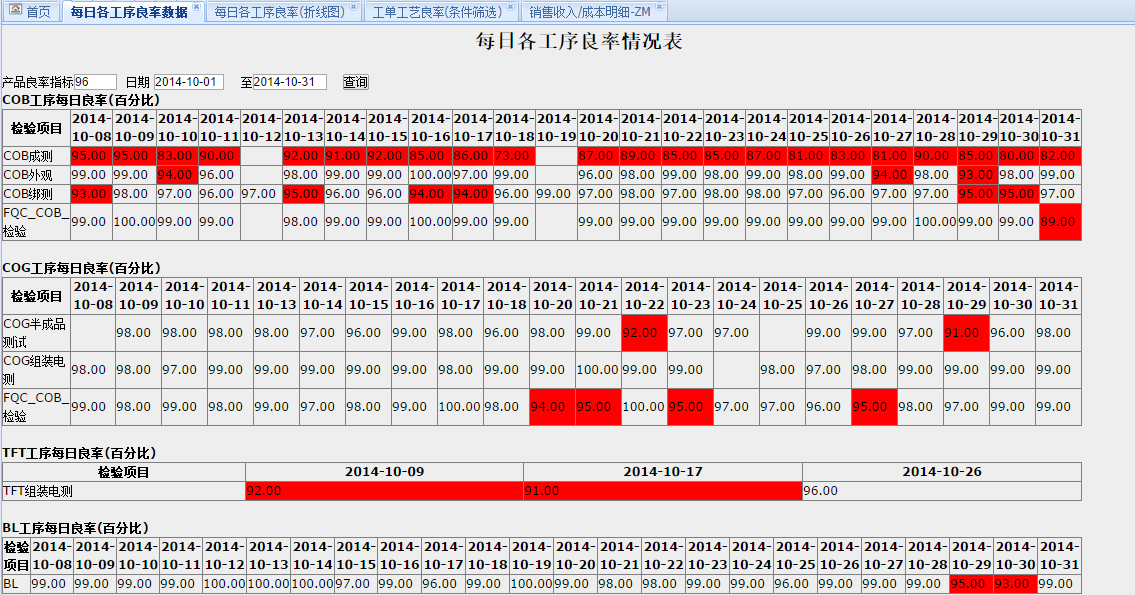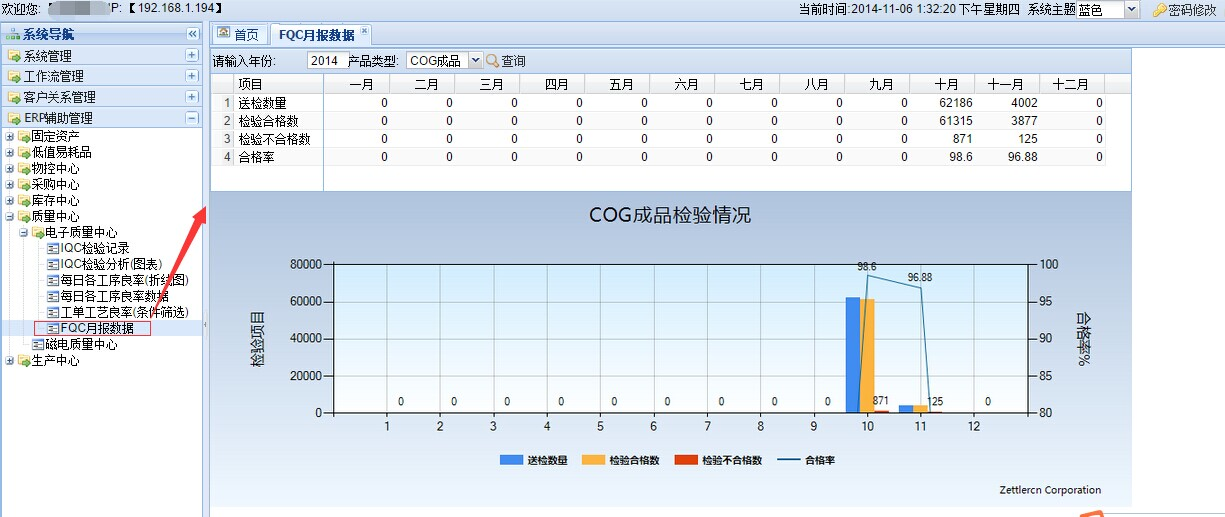# [易飞]简易制程日报-月报

1.撰写简易录入程序

数据来源ERP，由各条生产线班组长和QC录入。2.各工序统计图；3.日报折线图并不直观增加数据显示

动态列显示哟（根据任意日期区间纵向排列）

--抓取数据写入临时表#tempcob
select a.检验项目 , CONVERT(char(10),a.日期,120)日期,Convert(decimal(18,2),cast((SUM(a.测试数量)-SUM(a.不良数量)) as decimal(18,2))/sum(测试数量))*100  as 良率
into  #tempcob
from (select 日期,检验项目, 测试数量, 不良数量 from 制程COB成测 union all
select 日期,检验项目, 测试数量, 不良数量 from 制程COB外观 union all
select 日期,检验项目,测试数量, 不良数量 from 制程COB绑测 union all
select 送检日期,'FQC_COB_检验',检验数量,不合格数量 from 制程FQC_COB_检验
) as a
where CONVERT(char(10),日期,120)>='2014-10-01'  and CONVERT(char(10),日期,120)<= '2014-10-30'
group by a.检验项目,a.日期

--查看临时表数据，取分布日期（不重复）
--select   日期 from #tempcob
--select  distinct 日期 from #tempcob
DECLARE @SQL NVARCHAR(4000)=N'';
--这里使用了xml处理来处理类组字符串
SET @SQL=STUFF((SELECT N','+QUOTENAME(b.日期) FROM (select  distinct 日期 from #tempcob) as b
FOR XML PATH('')),1,1,N'');
--加入了xml处理和SQL注入预防判断
IF  dbo.fn_CheckSQLInjection(@SQL)=0
SET @SQL='select * from #tempcob pivot (max(良率) for 日期 in ('+@SQL+')) as tt'
EXEC(@SQL);
drop table #tempcob

--既然是用到了动态SQL,就有一个老话题:SQL注入。建一个注入性字符的判断函数。
CREATE FUNCTION [dbo].[fn_CheckSQLInjection]
(
@Col nvarchar(4000)
)
RETURNS BIT --如果存在可能的注入字符返回true,反之返回false
AS
BEGIN
DECLARE @result bit;
IF
UPPER(@Col) LIKE UPPER(N'%0x%')
OR UPPER(@Col) LIKE UPPER(N'%;%')
OR UPPER(@Col) LIKE UPPER(N'%''%')
OR UPPER(@Col) LIKE UPPER(N'%--%')
OR UPPER(@Col) LIKE UPPER(N'%/*%*/%')
OR UPPER(@Col) LIKE UPPER(N'%EXEC%')
OR UPPER(@Col) LIKE UPPER(N'%xp_%')
OR UPPER(@Col) LIKE UPPER(N'%sp_%')
OR UPPER(@Col) LIKE UPPER(N'%SELECT%')
OR UPPER(@Col) LIKE UPPER(N'%INSERT%')
OR UPPER(@Col) LIKE UPPER(N'%UPDATE%')
OR UPPER(@Col) LIKE UPPER(N'%DELETE%')
OR UPPER(@Col) LIKE UPPER(N'%TRUNCATE%')
OR UPPER(@Col) LIKE UPPER(N'%CREATE%')
OR UPPER(@Col) LIKE UPPER(N'%ALTER%')
OR UPPER(@Col) LIKE UPPER(N'%DROP%')
SET @result=1
ELSE
SET @result=0
return @result
END
GO4.月报数据